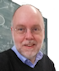Roland Potthast Webhome

misc3

Hello Jehan

Latex Writing works for example like this:

$\alpha$ is given by \alpha included in \$\$

or we can generate an equation by

\begin{equation}
\sum_{n=0}^{\infty} \beta_{n}
\end{equation}

leading to \begin{equation} \sum_{n=0}^{\infty} \beta_{n} \end{equation}

misc3.txt · Last modified: 2015/10/17 18:48 (external edit)

Page Tools